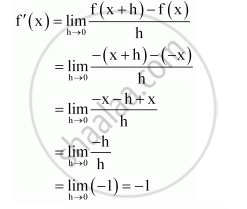Share

# Find the Derivative of the Following Functions from First Principle: −X - Mathematics

#### Question

Find the derivative of the following functions from first principle:

−x

#### Solution

Let f(x) = –x. Accordingly,f(x + h)  = -(x +h)

By first principle,Is there an error in this question or solution?

#### APPEARS IN

NCERT Solution for Mathematics Textbook for Class 11 (2018 (Latest))
Chapter 13: Limits and Derivatives
Q: 1.1 | Page no. 317

#### Video TutorialsVIEW ALL 

Solution Find the Derivative of the Following Functions from First Principle: −X Concept: Introduction of Derivatives.
S